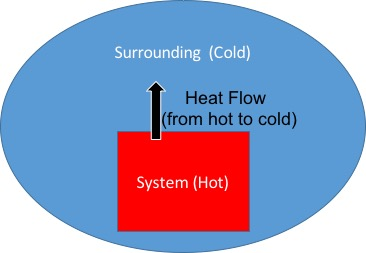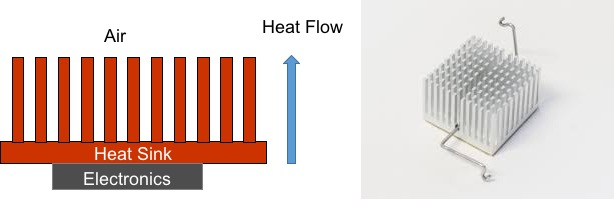# Air Cooled Heat Exchanger: Definition & Design

Instructor: Raghav Mahalingam

Raghav has a graduate degree in Engineering and 20 years of professional experience.

This lesson describes the working principle and design of an air cooled heat exchanger. The fundamental heat transfer equation used for an air-cooled heat exchanger is described and is used to explain the design methodology for designing an air-cooled heat exchanger.

## Heat Exchange Basics

Imagine going for a run on a hot day. During the run, your body temperature goes up. One of the ways to cool yourself down is by standing in front of a fan. The process of lowering our body temperature using airflow from a fan is called air cooling.

Similarly, heat energy is produced during the operation of all mechanical and electronic systems. Heat is usually considered a waste product and must be removed from the system to ensure reliable operation. The process of moving heat from one point to another is called heat transfer and the device used for the process of heat transfer is called a heat exchanger.

## Heat Transfer Principle

Heat transfer (or heat flow) always occurs from a hotter temperature point to a colder temperature point. Therefore, when heat has to be removed from a system, it has to be transferred to a region of space that is cooler than the system itself. For many engineering systems, the local air surrounding the system is the most convenient region to remove the heat to, since air is present everywhere, is free and in most cases, cooler than the operating system itself (See Figure 1). There are exceptions where the heat may be moved to a cooled liquid or even to the earth, but for this lesson we will not consider those situations.The amount of heat flow from a hot system to cooler surrounding air (or ambient air) depends on three factors:

1. The temperature difference between the system and the ambient air
2. The area of the system exposed to the ambient air.
3. A parameter called the heat transfer coefficient which depends primarily on the flow speed of the ambient air around the system.

This is expressed in equation form as Q = h x A x DT, where Q is the amount of heat transferred per unit time, h is the heat transfer coefficient, and A is the area of the system exposed to the air, and DT is the temperature difference. Therefore, it can be seen the the heat transfer rate increases with increasing the area exposed to air.

## Heat Exchangers

A heat exchanger serves two purposes:

1. Move that heat from inside a system to a surface that is exposed to ambient air. This process is called conduction heat transfer.
2. Move the heat from the surface to the ambient air. This process is called convection heat transfer and this is where the increased surface area matters.

The figure below shows a schematic and an example of a simple heat exchanger (usually called a heat sink) used to cool electronic chips. The little pin like array of structures in the figure are called fins and they serve to increase the surface area of the heat exchanger. In many situations a fan may be used to blow air over the heat sink to enhance the rate at which heat is removed from the system. Increasing air flow increases the heat transfer coefficient, h, and therefore increases the heat transfer rate.To unlock this lesson you must be a Study.com Member.

### Register to view this lesson

Are you a student or a teacher?